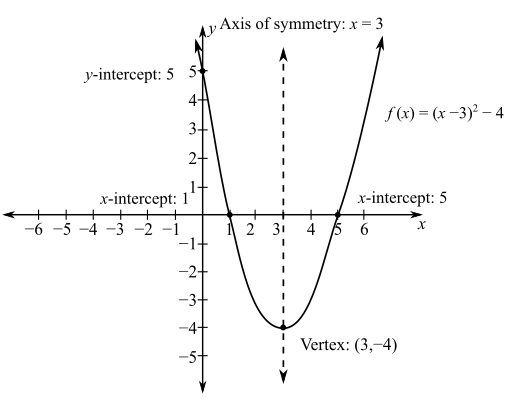# The graph of the given quadratic function and also, the domain and range of the function.BuyFind

### College Algebra, Books a la Carte ...

6th Edition
Robert F. Blitzer
Publisher: PEARSON
ISBN: 9780321869357BuyFind

### College Algebra, Books a la Carte ...

6th Edition
Robert F. Blitzer
Publisher: PEARSON
ISBN: 9780321869357

#### Solutions

Chapter 3, Problem 1MCCP
To determine

Expert Solution

## Answer to Problem 1MCCP

Solution: The range of function f is [4,) and the domain of the function is the set of all real number or (,).### Explanation of Solution

Given: The function is f(x)=(x3)24.

Comparing the provided equation f(x)=(x3)24 to the standard parabolic equation f(x)=a(xh)2+k.

Where, a=1,h=3,k=4.

The vertex of the parabolic equation is (h,k)=(3,4).

Determine the x intercepts.

Put f(x)=0.

f(x)=(x3)240=(x3)24x3=±2

This implies that:

x3=±2

For +2

x3=2x=5

For 2

x3=2x=1

The x intercept are 5 and 1. And the parabola passes through the points (5,0) and (1,0).

Now, to find the y intercept, put x=0.

f(x)=(x3)24=(03)24=94=5

The y intercept is y=5 and parabola passes through the point (0,5).

The graph of the parabola equation is shown below as:Now, compute the domain.

The x axis on the graph corresponds to the domain of the function. In the graph it is clear that the domain of the function is the set of all real number or (,).\

The range of the function is the output on the y axis on the graph. Since, the vertex is -4, the points on y axis fall at or above -4. Thus, the range of the function is [4,).

Conclusion: The graph shows that the parabola’s vertex (3,4) is the lowest point. Thus, the range of function f is [4,) and the domain of the function is the set of all real number or (,).

### Want to see more full solutions like this?

Subscribe now to access step-by-step solutions to millions of textbook problems written by subject matter experts!

### Want to see more full solutions like this?

Subscribe now to access step-by-step solutions to millions of textbook problems written by subject matter experts!© 2021 bartleby. All Rights Reserved.